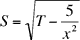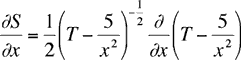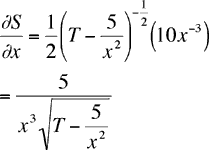Quandaries and Queries Is it possible to differentiate the following equation, if so could you please explain. S=SQRT(T-(5/X^2)) I would like the derivative of S with respect to X. I have had a go at this myself and arrived at the 5/(x^2*(sqrt(t*x^2-5)) Regards David Hi David, I think some symbols didn't survive through the email so let me make sure I have the correct problem. You want the partial derivative of S with respect to x whereUsing the chain rule I getorHarley Go to Math Central